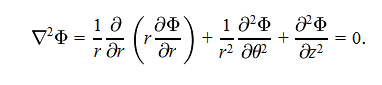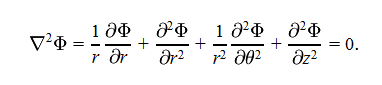# Solving Laplace Eqn by Separation of vbls

• Vapor88

#### Vapor88

Okay, I'm stumped at what seems like a very simple mathematical stepThen, the next step isI see what changed, but I don't understand exactly what happened. Can someone please explain? Thanks in advance!

They just applied the product rule:

$$\frac{\partial}{\partial x} \left(f(x)g(x)\right) = g(x) \frac{\partial f(x)}{\partial x} + f(x) \frac{\partial g(x)}{\partial x}$$

*facepalm*

Thanks. Also, just realized this is in the wrong forum. I'm on a roll...